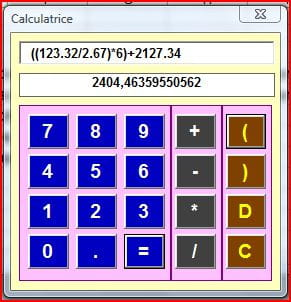4
Thanks

A few words of thanks would be greatly appreciated.

# Excel VBA Calculator

Although Microsoft Excel itself is a big calculator, using Excel Macro & VBA remains a popular method to create a calculator. Obviously, lots of coding is required to build a prototype of a calculator and it can only run after a full proof debug has been performed. The article below takes you through the steps necessary to build a calculator feature in Excel VBA.## How to Create a Calculator in Excel VBA

Below is the coding to create a Calculator feature in Excel VBA.

Highlights:

• Class Module
• UserForm
• Collection of Classws
• Collection of objects